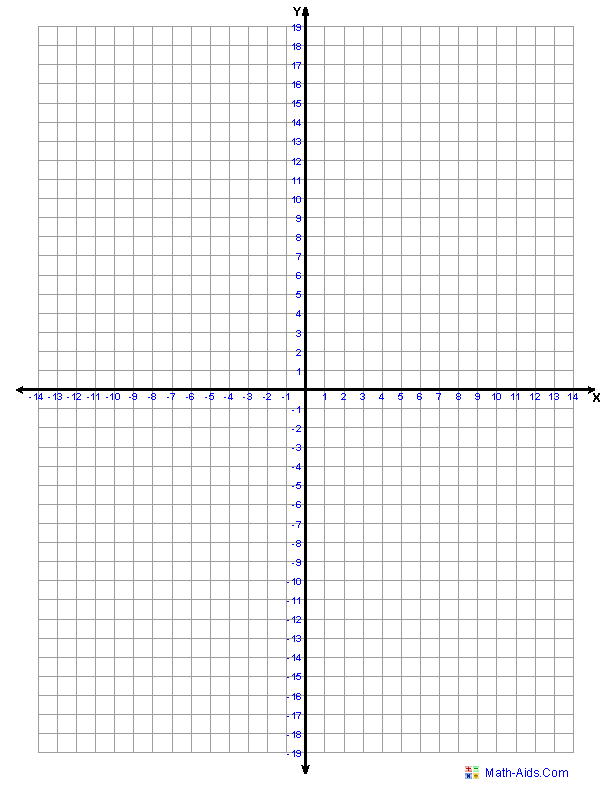# [Tex/LaTex] How to generate a simple cartesian plane system

plottikz-pgf

I need to make a cartesian plane for plotting the straight line joining the points (-3,1) and (6,-2). This is a very simple cartesian plane plotting. When I looked at the manual for tikz the graphics for cartesian planes are for more advance plotting, and what I need is very simple.

Something like this:A pstricks solution. The pst-eucl package is designed for plane geometry. Its \pstlineAB command defines a line with two points; the line can stop at a chosen distance of the two points, wich allows for lines defined by two pointsthat are figure-wide (too long lines are cropped by the pspicture* environment). I had to patch the labels-making command in order to use coloured sans maths as in the figure.

\documentclass[a4paper, 11pt, x11names, svgnames]{article}
\usepackage[margin=1.5cm]{geometry}
\usepackage{auto-pst-pdf}
\usepackage{xcolor}

\usepackage{sansmath}
\usepackage{etoolbox}
\pretocmd{\pshlabel}{\color[rgb]{0.27 0.32 0.71}\sansmath}{}{}
\pretocmd{\psvlabel}{\color[rgb]{0.27 0.32 0.71}\sansmath}{}{}{}%

\begin{document}%

\psset{unit=5mm, ticks=none, xlabelsep=1pt, ylabelsep=1pt}

\begin{pspicture*}(-15,-20)(15,20)
\psaxes[labelFontSize = \scriptstyle\sansmath]{<->}(0,0)(-15,-20)(15,20)[\textsf{X}\rule{0pt}{2.25ex},-120][\textsf{Y}, -150]
\psset{linecolor=DodgerBlue4, tickcolor=white, subtickcolor=DodgerBlue4, gridlabelcolor=Dodgerblue4, ,linewidth=1.2pt}%
\pstGeonode[PosAngle=90](-3,1){A}(6,-2){B}
\pstLineAB[linecolor=SlateGray3, nodesep=-20]{A}{B}
\ncline[offset=-1.2pt, linecolor=LightSalmon1]{A}{B}
\psset{gridwidth=0.3pt, subgriddiv=1,gridlabels=0pt}
\psgrid(-14,-19)(14,19)
\end{pspicture*}

\end{document}